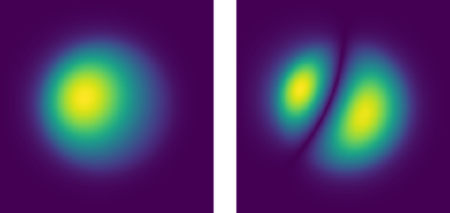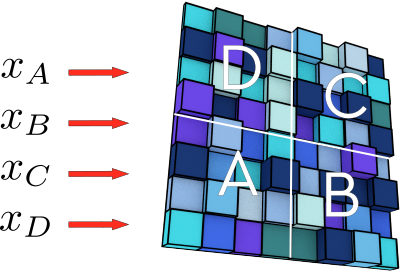Created by sebastien.popoff on 26/05/2019

## Compare Different Methods of Modes Estimation of Bent Multimode Fibers with pyMMFIn a previous tutorial, I explained how to calculate the modes of a bent multimode fibers. I introduced two methods, following the approach published in [M. Plöschner, T. Tyc, and T. Čižmár, Nat. Photon. (2015)]. In this short tutorial, I show how to use pyMMF to simulate bent fibers and compare the two different methods. A Jupyter notebook can be found on my Github account: compare_bending_methods.ipynb.

Created by sebastien.popoff on 15/04/2019

## Wavefront shaping in complex media for analog computation[M. W. Matthès et al., Optica, 6 (2019)]

Performing linear operations using optical devices is a crucial building block in many fields ranging from telecommunications to optical analog computation and machine learning. For many of these applications, key requirements are robustness to fabrication inaccuracies, reconfigurability, and scalability. Traditionally, the conformation or the structure of the medium is optimized in order to perform a given desired operation. Since the advent of wavefront shaping, we know that the complexity of a given operation can be shifted toward the engineering of the wavefront, allowing, for example, to use any random medium as a lens.

Created by sebastien.popoff on 25/02/2019

## Part 1: Step Index Benchmark

I recently published a two-part tutorial on how to find the modes of an arbitrary multimode fiber without or with bending. Based on this tutorial, I published a (still experimental) version of a Python module to find the modes of multimode fibers and calculate their transmission matrix: pyMMF. The goal of this module is not to compete with commercial solutions in terms of precision but to provide a way to easily simulate realistic fiber systems. To validate the approach, I use step-index multimode fibers as a benchmark test as the dispersion relation is analytically known (see my tutorial here) and for which the Linearly Polarized (LP) mode approximation yields good results. I focus my attention here on the precision of the numerically found propagation constants.

Created by sebastien.popoff on 29/12/2018

## Part 2: Bent Fibers

We saw in the first part of the tutorial that the profiles and the propagation constants of the propagation modes of a straight multimode fiber can easily be avulated for an arbitrary index profile by inverting a large but sparse matrix. Under some approximations , a portion of fiber with a fixed radius of curvature satisfies a similar problem that can be solved with the same numerical tools, as we illustrate with the PyMMF Python module . Moreover, when the modes are known for the straight fiber, the modes for a fixed radius can be approximate by inverting a square matrix of size the number of propagating modes . It allows fast computation of the modes for different radii of curvature.